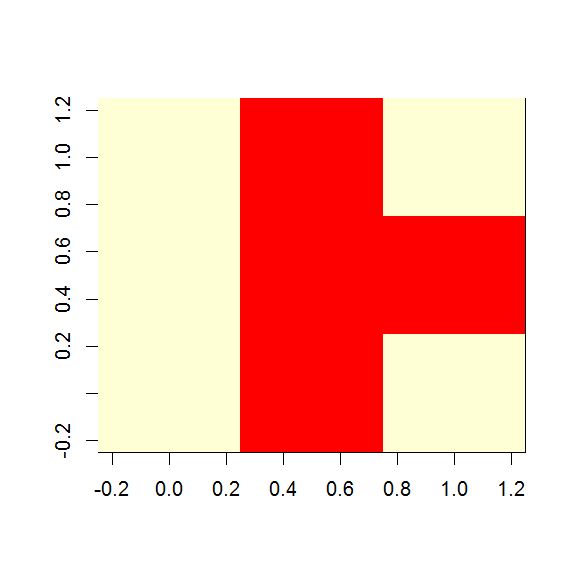# Creating an image of a matrix in R using image()

October 6, 2016
By

(This article was first published on R – Opiniomics, and kindly contributed to R-bloggers)

You have a matrix in R, and you want to visualise it – say, for example, with each cell coloured according to the value in the cell.  Not a heatmap, per se, as that requires clustering; just a simple, visual image.

Well, the answer is image() – however, there is the slightly bizarre coding choice buried in the help:

Notice that `image` interprets the `z` matrix as a table of `f(x[i], y[j])` values, so that the x axis corresponds to row number and the y axis to column number, with column 1 at the bottom, i.e. a 90 degree counter-clockwise rotation of the conventional printed layout of a matrix.

Let’s look at that:

``````

mat <- matrix(c(1,1,1,0,0,0,1,0,1), nrow=3, byrow=TRUE)
mat
[,1] [,2] [,3]
[1,]    1    1    1
[2,]    0    0    0
[3,]    1    0    1

image(mat)
``````

This produces:We can clearly see the 1,0,1 row is now the right-hand column i.e. the matrix has in fact been rotated 90 degrees anti-clockwise.

To counteract this we need to rotate the input matrix clockwise before passing to image:

``````
rotate <- function(x) t(apply(x, 2, rev))

image(rotate(mat))
``````

This now produces what we might expect:EasyR-bloggers.com offers daily e-mail updates about R news and tutorials on topics such as: Data science, Big Data, R jobs, visualization (ggplot2, Boxplots, maps, animation), programming (RStudio, Sweave, LaTeX, SQL, Eclipse, git, hadoop, Web Scraping) statistics (regression, PCA, time series, trading) and more...

If you got this far, why not subscribe for updates from the site? Choose your flavor: e-mail, twitter, RSS, or facebook...